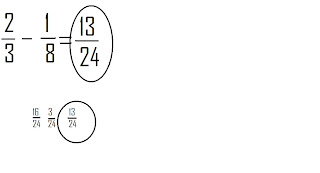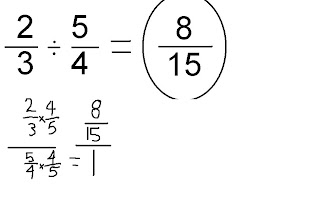### Casey's Last Fraction Post

Monday, May 11, 2009

When you add fractions the easiest way to do it is if you find the common denominators.Once you find the common denominator than you can add the numerators and fiond your answer.Adding Question 2:Subtracting Question 1:

When you subtract fractions the easiast way to do it is also find the common denominator.The common denominator helps you because you wont need to subtract the denominators anymore,only the numerators.Multiplying 1:

When multiplying Fractions all you need to do is multiply the denominators and then multiply the numerators.

4/6 x 2/4 = 8/24

4x2=8

6x4=24

Dividing Question 1:

When you Divide fractions the easiest way to do it is to use the Reciprical. The Reciprical is the fraction in reverse.Any time you use it, the answer should equal to one.Dividing Question 2:Dividing Question 3:Word Problem:
2) It takes 2 1/4 scoops of flour to make one cake. How many cakes do 15 scoops make?

15 scoops will make 6 1/2 cakes.